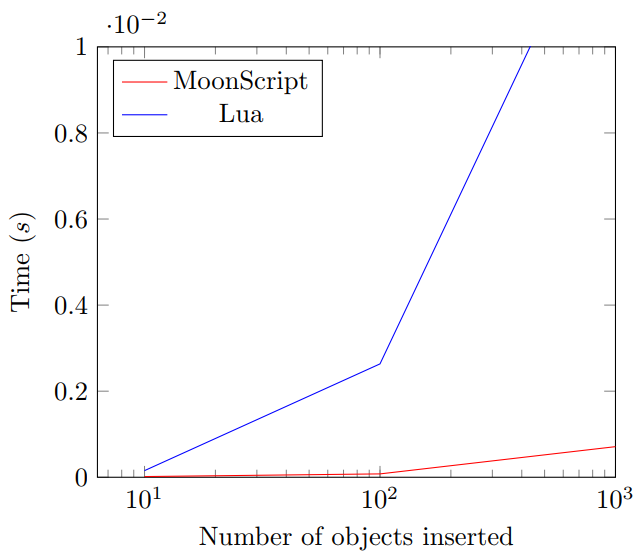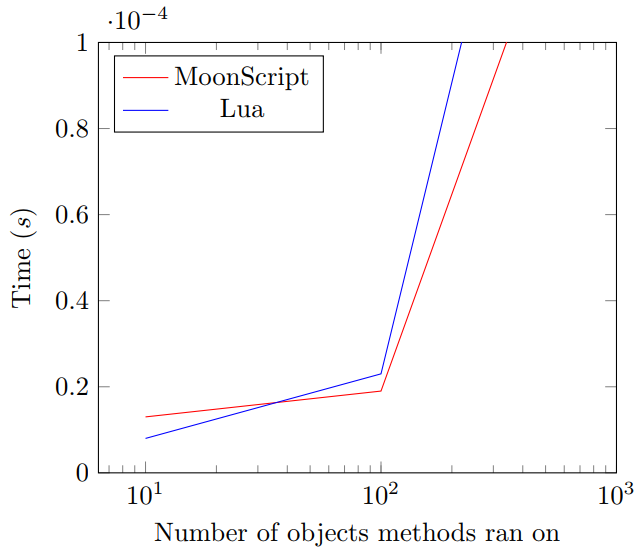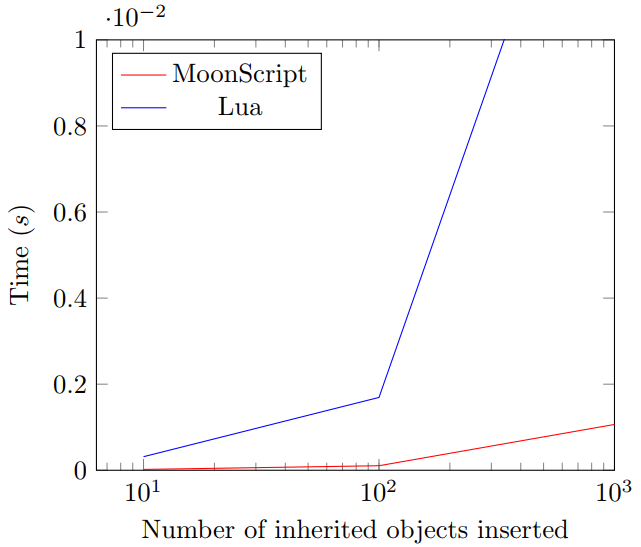# MoonScript vs Lua: A speed test

I've discovered MoonScript in the last month and so far I'm loving it (apart from a few whitespacing quirks). One of the things I like most about it is the built-in Object Orientation, something that is lacking from Lua (but can be added with libraries/metatables).

I've been interested in how fast the generated Lua code is from MoonScript for a while now, so today I'm going to do a little speed-test of sorts. This won't be an incredibly indepth comparison - so don't jump down my throat if I've fucked up somewhere. Feel free to correct me though.

I'll be comparing speed differences from MoonScript OO to a minimal and fast Lua OO library, 30log.

The main 3 pillars of Object Orientation are: Objects, Methods and Inheritance. I'll be seeing how long it takes each implementation to create these in increasing sizes.

## Creating objects

Simply defining a class called `X`, and creating and inserting ever-increasing amounts of `X`, from 10 to 1,000,000 instances.

``--lualocal class = require '30log't = {}X = class("X")Start = os.clock()for i=1, 100 do table.insert(t, X:new()) endEnd = os.clock()print(End-Start)``
``--moonscriptt = {}class X  new: () =>Start = os.clock()for i=1, 100 do table.insert(t, X!)End = os.clock()print(End-Start)````` | No. of objects created | Time taken (s) | |------------------------|------------|-----------| | | MoonScript | Lua | | 10 | 1.6e-05 | 0.000154 | | 100 | 7.8e-05 | 0.002634 | | 1,000 | 0.000711 | 0.014191 | | 10,000 | 0.006792 | 0.144497 | | 100,000 | 0.072799 | 1.493317 | | 1,000,000 | 0.708374 | 16.057709 | ```

As you can see, the time taken for 30log to create objects increases massively to 16 seconds! - Although realistically most people will have less than 1,000 objects, the speed difference is still pretty large.

## Methods

Giving `X` an method called `add` which simply increments its `self.x`.

``t = {}local class = require '30log'X = class("X")function X:init(x) self.x = x endfunction X:add() self.x = self.x + 1 endfor i=1, 10 do table.insert(t, X(0)) endStart = os.clock()  for i=1, #t do t[i]:add() endEnd = os.clock()print(End-Start)``
``t = {}class X  new: (@x) =>  add: () =>    @x += 1for i=1, 100000 do table.insert(t, X(0))Start = os.clock()for x in *t do x\add()End = os.clock()print(End-Start)````````| No. methods ran | Time taken (s)        |
|-----------------|------------|----------|
|                 | MoonScript | Lua      |
|-----------------|------------|----------|
| 10              | 1.3e-05    | 7.98e-06 |
| 100             | 1.9e-05    | 2.3e-05  |
| 1,000           | 0.000171   | 0.000248 |
| 10,000          | 0.001755   | 0.00275  |
| 100,000         | 0.017076   | 0.029891 |
| 1,000,000       | 0.172534   | 0.312667 |
``````

MoonScript is initially slower when object count is <100, but soon to lag starts to mount with 30log.

## Inheritance

Creating another class called `Y` that is inherited from `X`, we should expect similar results to the first test.

``local class = require '30log't = {}X = class("X")function X:init(x) self.x = x endfunction X:add() self.x = self.x + 1 endY = X:extend("Y")function Y:init(x) self.x = x endStart = os.clock()for i=1, 10 do table.insert(t, Y(0)) endEnd = os.clock()print(End-Start)``
``  t = {}  class X    new: (@x) =>    add: () =>      @x += 1  class Y extends X    new: () =>  Start = os.clock()  for i=1, 100000 do table.insert(t, Y(0))  End = os.clock()  print(End-Start)````````| No. of objects inherited | Time taken | (s)       |
|--------------------------|------------|-----------|
|                          | MoonScript | Lua       |
| 10                       | 1.9e-05    | 0.000315  |
| 100                      | 0.000105   | 0.001692  |
| 1,000                    | 0.001064   | 0.017323  |
| 10,000                   | 0.01217    | 0.165252  |
| 100,000                  | 0.109585   | 1.721108  |
| 1,000,000                | 1.042791   | 17.100362 |
``````

MoonScript is the clear victor here, 30log takes up to 17 seconds to complete the same task as MoonScript.

So yeah. I guess we can say MoonScript OO is faster then 30log OO (and also nicer looking). 30log is still a good OO library though, it provides a simple and fairly fast way to do OO programming without the need for pre-compiling.